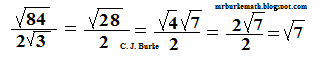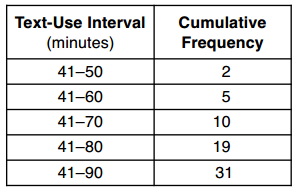## Saturday, May 10, 2014

### January 2014 Algebra Regents, Part II and III

Note: Part I of the test was covered in two earlier posts: 1 - 10 and 11-30.
You can also check out the Regents tag.

### Part II

31. Express (84)^.5 / (2 * (3) ^.5) in simplest radical form.
In other words: Express the square root of 84 divided by the product of 2 times the square root of 3, in simplest radical form.

Divide 84 by 3. Do NOT divide 84 by 2. There are Rules for Radicals, because square roots are numbers with the same exponent (i.e., one-half).
84 / 3 = 28, so this becomes the (square root of 28) / 2. Radical 28 can be factored into radical 4 times radical 7. However, the square root of 4 is 2. So you have 2 times the square root of 7 over 2. That simplifies into the square root of 7. Here's a picture:32. The cumulative frequency table below shows the number of minutes 31 students spent text messaging on a weekend.Determine which 10-minute interval contains the median. Justify your choice.

There are 31 pieces of data (in this case, students). The median is the amount of time spent by the 16th student because he or she is in the middle. (Think if they were lined up in order.) 41-70 is less than 15, but 41-80 is over 15, so the 15th student is in the 71-80 group. Note: If you said 41-80, you would have lost a point because it specifically says that it must be in the ten-point interval.

33. Kirsten invested \$1000 in an account at an annual interest rate of 3%. She made no deposits or withdrawals on the account for 5 years. The interest was compounded annually. Find the balance in the account, to the nearest cent, at the end of 5 years.

Use the Compound interest formula. If you don't remember the compound interest formula, use I=PRT to figure out the interest for the first year. Add the interest to the \$1000 principal, and use the formula on the new value for the second year. Repeat until you have all five years. This is acceptable. It is a lot of work for two points, but it is acceptable.

The actual formula you should be using is B = P(1 + (r/n))nt, where n is the number of times per year that you are compounding interest. Since it's only one time per year (annual rate), n = 1 and it falls out of the formula.
Substitute and you get B = 1000(1 + .03)5 = 1159.274, which rounds down to \$1,159.27.

If you think you might be wrong with the formula, use the first method.

### Part III

34. Graph and label the functions y = |x| and y = |2x| on the set of axes below. Explain how increasing the coefficient of x affects the graph of y = |x|.

The absolute value means that y is equal to the value of x or the value of 2x without the sign (i.e., positive). You will have two V-shaped graphs with their bottoms on the origin (0, 0). (I'm not really sure how much more I can say about this without just posting a graph.)

Explain: Increasing the co-efficient of x will make the graph more narrow.

35. Terry estimated the length of the edge of a cube to be 5 cm. The actual length of the side is 5.2 cm. Find the relative error of the surface area of the cube, to the nearest thousandth.

Relative error -- one of my least favorite topics because a) it doesn't lead into another topic in any meaningful way, and b) if you express the answer as a percent, which you were required to do just a few years ago, you will lose a point. Seriously.

The Surface Area of a Cube is six times the area of one face, which equals six times an edge squared: 6s2.
Relative error is the difference in the two surface areas, divided by the actual surface area. So ((6)*(5.2)^2-(6)*(5)^2)/((6)*(5.2)^2)=0.075443787, which is 0.075 to the nearest thousandth. Again, rounding errors will cost you a point. Don't make them.

36. From the top of an apartment building, the angle of depression to a car parked on the street below is 38 degrees, as shown in the diagram below. The car is parked 80 feet from the base of the building. Find the height of the building, to the nearest tenth of a foot.

The angle of depression is the same as the angle of the triangle where the car is. The wall is opposite the car. The ground is adjacent to the car. This a trigonometry question involving the tangent. That is, Tan 38 degrees = x / 80.
So x = 80 * Tan 38 degrees, which is 80 * 0.781285627 = 62.50285012, if I've calculated correctly, which is 62.5 feet.

to be continued . . .Anonymous said...
This comment has been removed by a blog administrator.
(x, why?) said...

Anonymous wrote the following:

the [HECK]? i still dont get ur explenations, they're amd unclear. esp/ the last one..guhh its so confusing like, i thought it was cos. how the helll is is tan. mad confusing----

(x, why?) said...

If you need someone to elaborate on any explanation, you need only to ask. Here's a tip: try being polite and you'll have more success with either me or one of my readers responding to your question.

As to the final question, I wrote: The wall is opposite the car. The ground is adjacent to the car.

Opposite and adjacent mean that we have to use tangent.

The line from the top of the building to the car would be the hypotenuse. It is not given and they do not ask for it. So we wouldn't use either sin or cos because both of them use the hypotenuse.

I hope this clears it up. If you have any further questions, please feel free to ask.

Nicely.